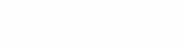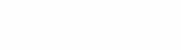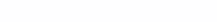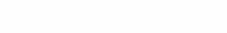# Comparator multivibrator

## Background

Once I needed to tinker with power electronics and needed a square-wave generator with an output voltage of about 24V and an oscillation frequency of 50..100 kHz. It was difficult with the equipment and, as always, I had to do with the means at hand.

The simplest solution is to make a 2-transistor multivibrator. But this option is bad, firstly, the transistor multivibrator does not give pulses with steep edges, which means that if it controls the power switches of the converter, then large switching losses will arise in them. Secondly, the oscillation frequency of the multivibrator is highly dependent on the supply voltage.

This happens because when the multivibrator is switched, a blocking voltage is applied to the base-emitter junction of the closed transistor, which is almost equal to the supply voltage.

So, the base of the transistor gets -24V, and the breakdown voltage of the transition is -5 ..- 10V. Naturally, when switching, an avalanche breakdown of the transition occurs. The multivibrator, of course, will work, but its reliability is low, and the oscillation frequency strongly depends on the supply voltage.

## The solution of the problem

What to do? Any operational amplifier can withstand 24V, so you can make a multivibrator on an op-amp, or even better on a comparator. The comparator is specially designed to obtain the maximum switching speed. A circuit of such a multivibrator can be found in any electronics textbook, for example, in  or …

How does it work? We look at the picture. The circuit has an RC charge-discharge circuit and a controlled voltage divider R1, R2, R3. The voltage at the divider is controlled by the voltage at the output of the comparator. If the output of the comparator is a logical unit, then it is equal to V1, and if a logical zero, then V0.

How does the circuit work? Let’s supply food. At the initial moment of time, the capacitor C has not yet been charged, and the voltage across it and at the inverting (minus) input of the comparator is zero. On the non-inverting (positive) input, the voltage is greater than zero. Therefore, the output of the comparator will be a logical unit, i.e., the voltage is almost equal to the supply voltage Vs (if Rn is much less than R3).

The voltage at the “positive” input V1 sets the divider, the upper arm of which is formed by resistors R1 and R3, connected in parallel to the source Vs, and in the lower arm is resistor R2.

Capacitor C is gradually charged by the output voltage of the comparator. As soon as the voltage across the capacitor reaches the voltage V1, the comparator switches over and a logic zero appears at its output.

The voltage at the controlled divider will decrease to a value V0 (now in the upper arm there is a resistor R1, and in the lower one, R2 and R3 are connected in parallel). Capacitor C2 will start discharging. As soon as the voltage across it reaches the voltage V0, the comparator will jump again and then the cycle of oscillations will be repeated.

## Period calculation

Now you can calculate the oscillation period of the multivibrator.

According to Ohm’s law, the voltage V1 is:We transform this formula in terms of conductivity:

V1 = Vs / (1 + R1 || R3 / R2) = Vs / (1 + y2 / (y1 + y3)) = Vs (y1 + y3) / (y1 + y2 + y3)

If all resistances are equal, then V1 = (2/3) Vs

Then we find the voltage V0 it is equal to:We transform this formula in terms of conductivity:

V0 = Vs / (1 + R1 / R2 || R3) = Vs / (1 + (y2 + y3) / y1) = Vs y1 / (y1 + y2 + y3)

If all resistances are equal, then V0 = Vs / 3

When the comparator switches, the capacitor C is discharged exponentially. The discharge time is equal to the charge time and is equal to half the period. Then:From here T = 2 RC ln (V1 / V0) = 2 RC ln[ ( y1 + y3 ) / y1 ]

Finally, the multivibrator period is:In a particular case, if R1 = R3 period T = 1.386 RC

If R3 = 2 R1 period T = 0.811 RC

## Practice

After this deep research, it’s time to get down to business 🤠. We take the most common comparator LM311. Its supply voltage limit is 36 V. It is suitable. The limiting output current is 50 mA. We choose the load resistance of 2 kOhm, while the current through it is 12 mA, and the allocated power is 144 mW, which is quite acceptable. Resistances R1 – R3 should be tens of kilo-ohms, and R3 is at least an order of magnitude greater than Rn. We choose their values ​​equal to 20 kOhm. The capacitance of the capacitor is C = 2200 pF.

In order not to waste time on the PCB layout, I used a reusable breadboard. The resulting experimental setup is shown in Figure 3.

It can be seen that the tops of the pulses are slightly “skewed” due to the capacitor charging current. The oscillation frequency turned out to be 14.12 kHz, and the calculated 16.4 kHz, the discrepancy is almost 14%, which is clearly more than 5% of the element tolerance. Why it happens? To figure it out, take a few capacitors that come to hand and measure how the frequency changes from capacitance.

 Capacitor capacity, pF Dielectric type Oscillation frequency, kHz Own mounting capacity — 1350 47 K10-17b imp, NP0 486 680 K10-17b imp, NP0 48.26 1000 K73-17, NP0 35.06 2200 K10-17b imp, X7R 14.12

The maximum possible oscillation frequency is determined by the intrinsic capacitance of the comparator and the mounting Cm and is equal to 1350 kHz. From this it is easy to calculate that Cm = 27 pF. The pulse fronts are shown in Figure 4 on the left, their shape is heavily overwhelmed. Quite steep edges can be obtained at frequencies below 500 kHz.

Let’s compare theory with practice, taking into account the mounting capacity.

 Capacitance, pF Theoretical frequency, kHz Practical frequency, kHz Error,% 27 1350 1350 0 47 + 27 487.5 486 -0.3 680 + 27 51.025 48.26 -5 1000 + 27 35.13 35.06 -0.1 2200 + 27 16.2 14.12 -12.8

It is now clear that the whole point is in the dielectric. The dielectric constant of X7R ceramics, and especially Y5V, is highly dependent on voltage and temperature. Therefore, capacitors with such a dielectric should not be used in frequency setting and integrating circuits.

Of course, instead of LM311, you can use similar domestic comparators 521СА3 or 554СА3, in this circuit they work no worse …

It became interesting, but how will the circuit behave when the temperature changes?

To find out, I made another layout. High-precision resistors were taken, such as C2-29V 0.1% R1-R3 = 30.1 kOhm, R = 37.5 kOhm, and capacitor C of the K73-17 brand with a value of 0.1 μF.

Settlement period schemes T = 1.386 RC = 5.2 ms corresponds to a frequency of 192.4 Hz.

 Temperature, ℃ Frequency Hz Deviation,% +85 191.4 -1.2 +25 193.7 0 -40 200.9 +3.7

The maximum frequency deviation in the industrial temperature range was almost 4%. But it can be reduced by using a highly stable capacitor K71-7, as well as microcircuits with low input currents and temperature drift of the bias voltage.

#### Literature.

1. P. Horowitz. W. Hill. The art of circuitry: Per. from English – Ed. 7th. – M .: Mir, BINOM, 2011 .– 704c. ill. / Page 321.

2. G.I. Volovich. Circuitry of analog and analog-digital electronic devices. 2nd ed., Rev. – M .: Dodeka, 2007 .– 528c. ill. / Page 197.

3. B. Uspensky. Integrated voltage comparators // To help radio amateurs: Collection. Issue 97 / Compiled by B.G. Uspensky – M.: DOSAAF, 1987. – 78. with ill. / Page 49.